# LLC 的工作原理（第 II 部分）：LLC 变换器设计考量## 概述

LLC 变换器的设计涉及众多的设计决策与关键参数，而且很多因素相互关联。任何一个设计选择都可能影响系统中的许多其他参数。LLC 谐振腔的设计是其中最大的挑战，因为它决定了变换器响应负载、频率和电压变化的能力。因此，设计人员必须正确定义变换器负载和频率的工作范围，因为这些值会影响谐振腔的值与参数来。

## LLC变换器增益

$$M_G(Q,L_N,f_N) = \frac {V_{OUT(AC)}}{V_{IN(AC)}} = \frac {f_N^2 \times (L_N-1)} {(f_N^2 -1)^2 + (f_N^2 \times (f_N^2 -1) \times (L_N-1)^2 \times Q^2}$$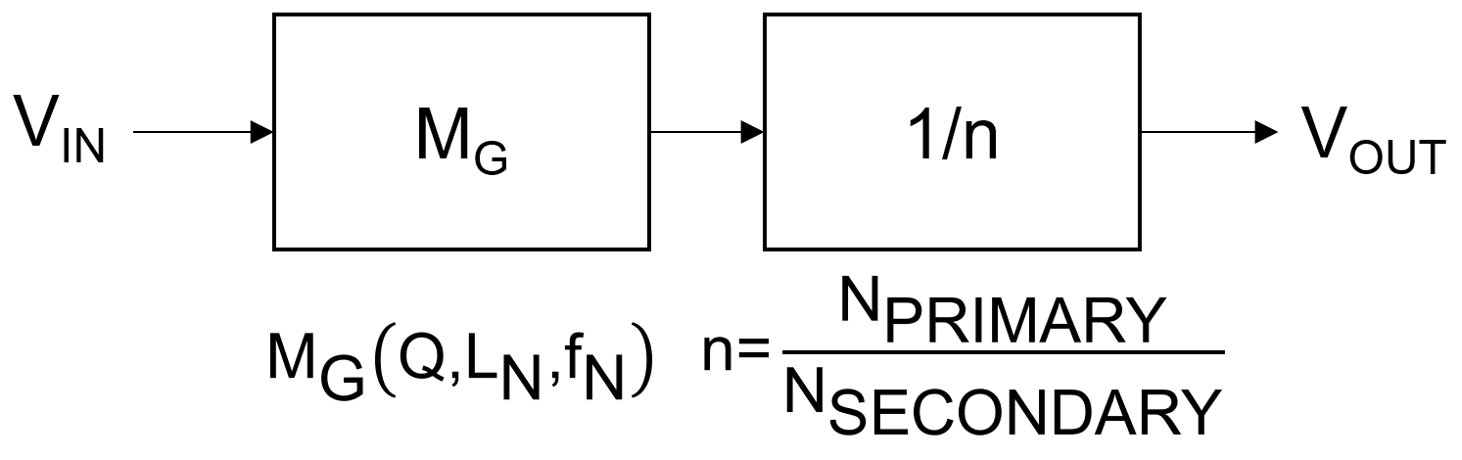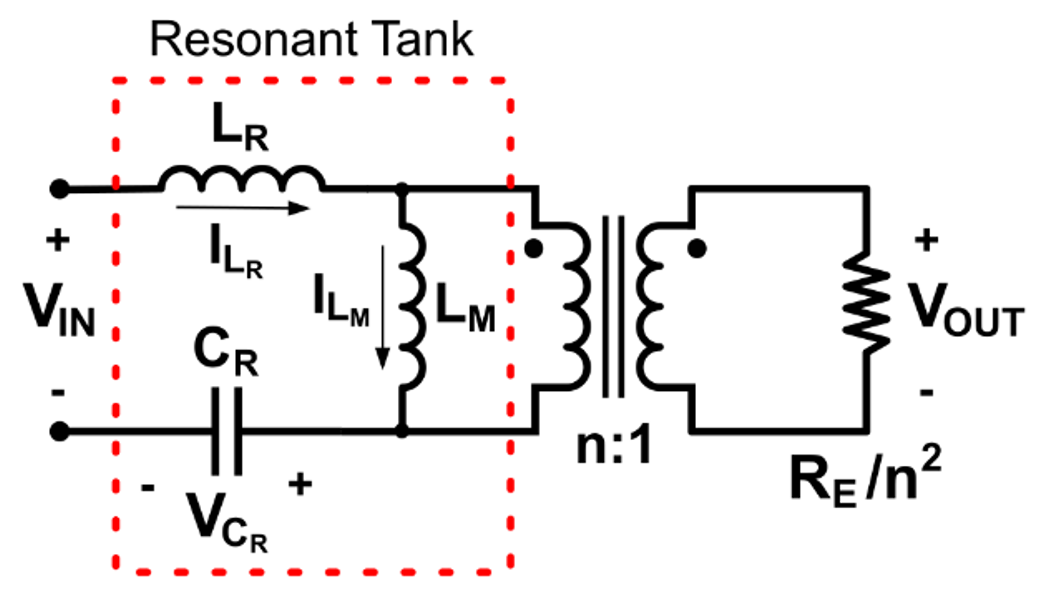$$\frac {V_{OUT}}{V_{IN}} = M_G \times \frac {1}{n}$$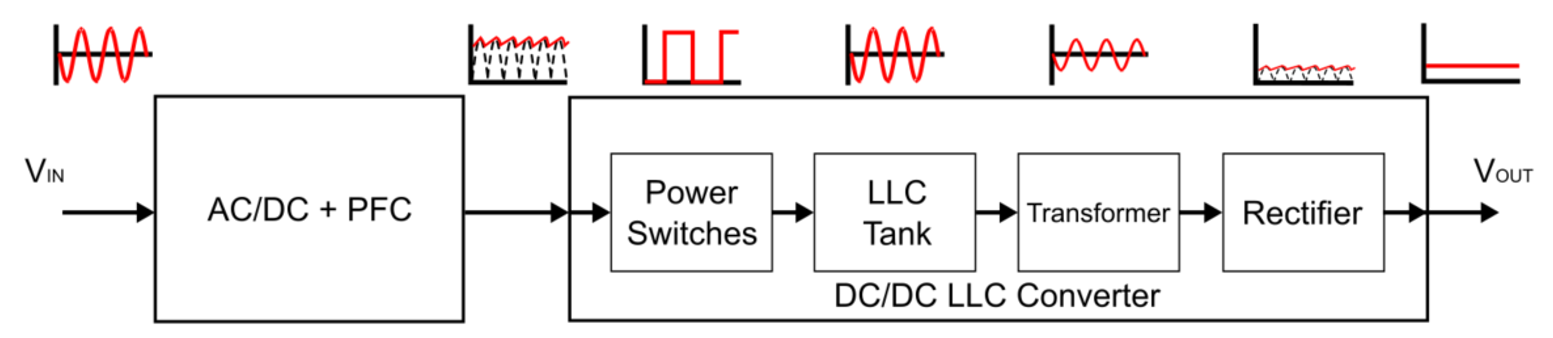AC/DC + PFC 级将 AC 输入电压 (VIN) （例如来自 AC 电源的功率）转换为稳定的 DC 电压，同时还保持输入电流与 VIN 同相。PFC 级对确保设计符合国际标准（包括 ISO、UNSCC、IEEE 和 CISPR）规定的各项功率因数规范十分必要。AC/DC + PFC 级的输出电压 (VOUT) 在理想情况下是稳定的，但由于组件的非理想化， AC/DC 输出端往往会出现电压纹波，这通常是寄生电感和电容ESR导致的，这种电压纹波也会出现在 LLC 变换器的输入端。

$$M_{G\_NOM} = \frac {V_{OUT} \times n} {V_{IN\_NOM}}$$

$$M_{G\_MAX} = \frac {V_{OUT} \times n} {V_{IN\_MIN}}$$

$$M_{G\_MIN} = \frac {V_{OUT} \times n} {V_{IN\_MAX}}$$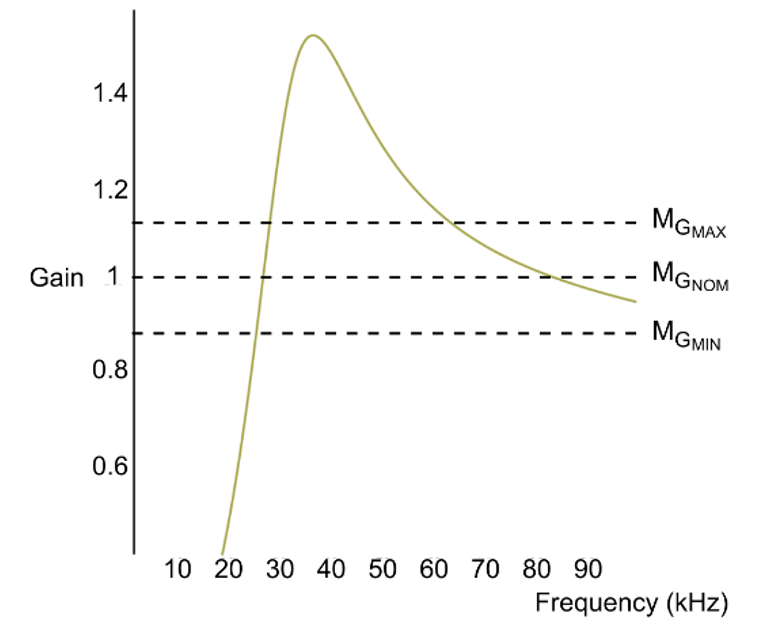## LLC变换器负载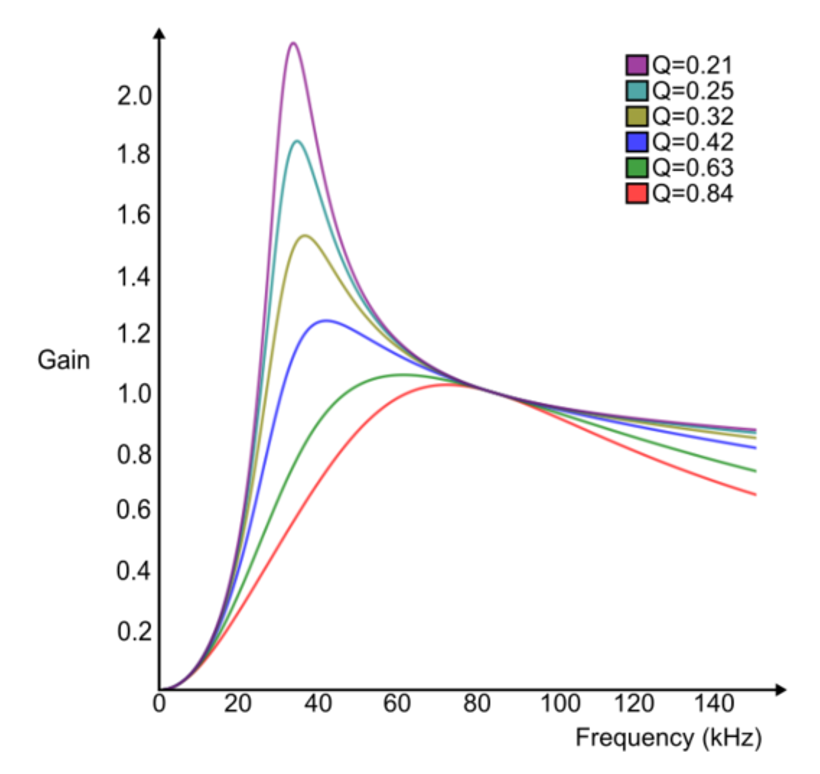## LLC变换器开关频率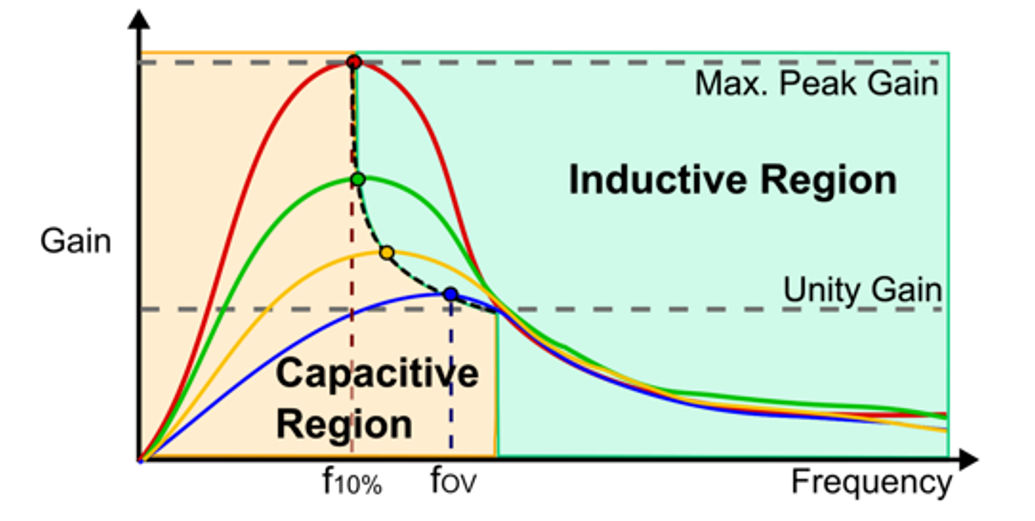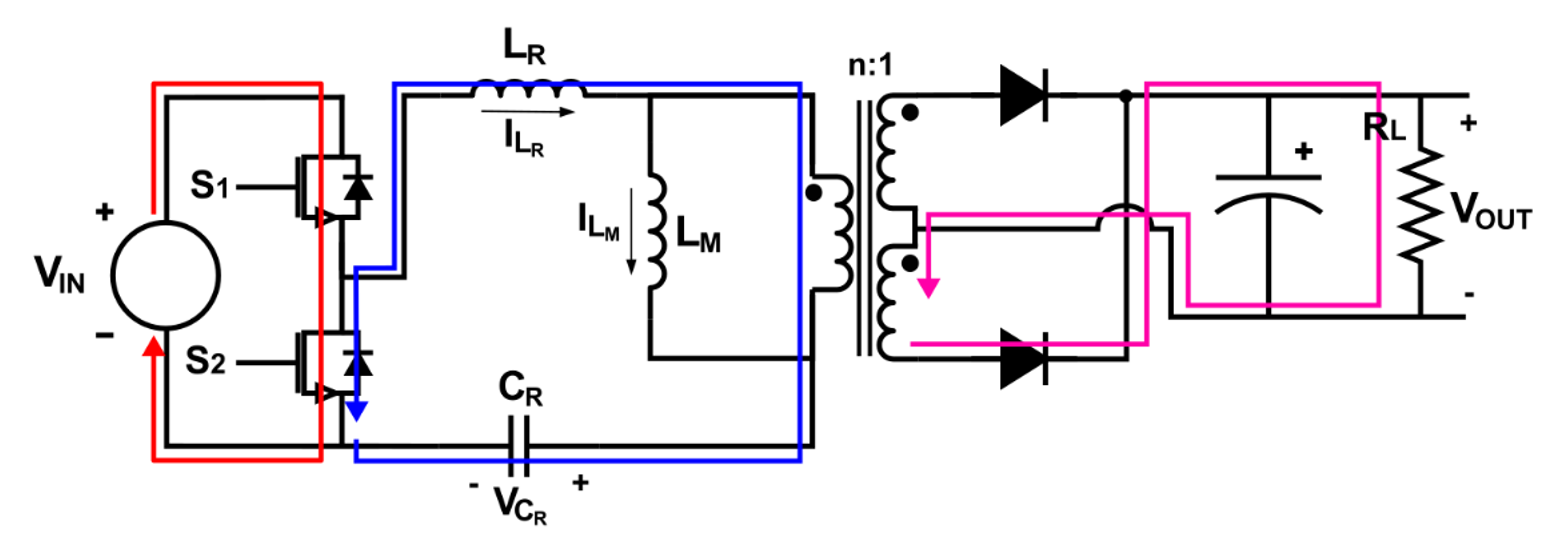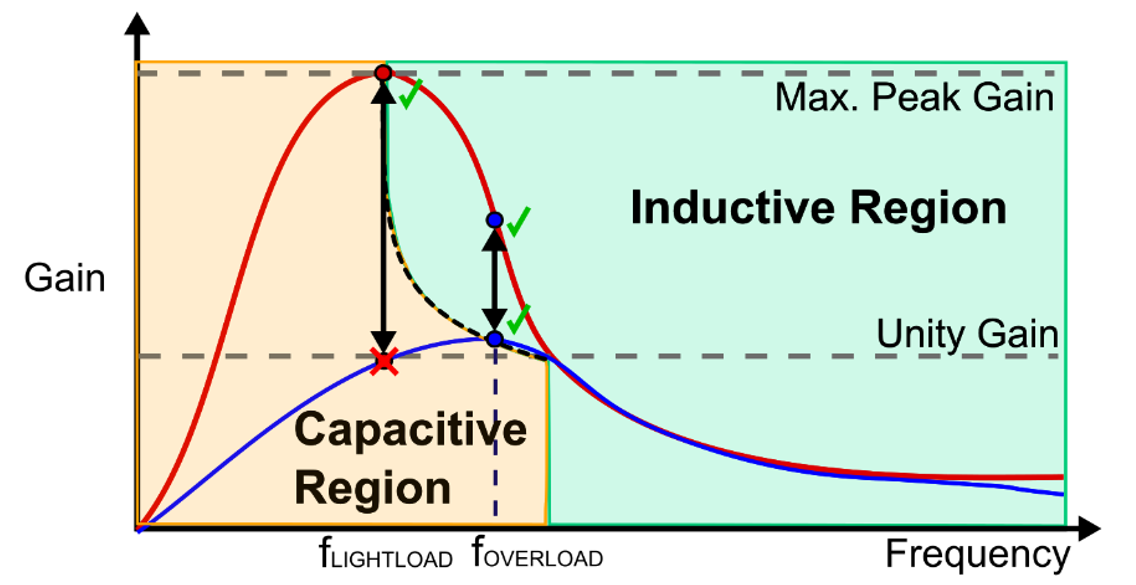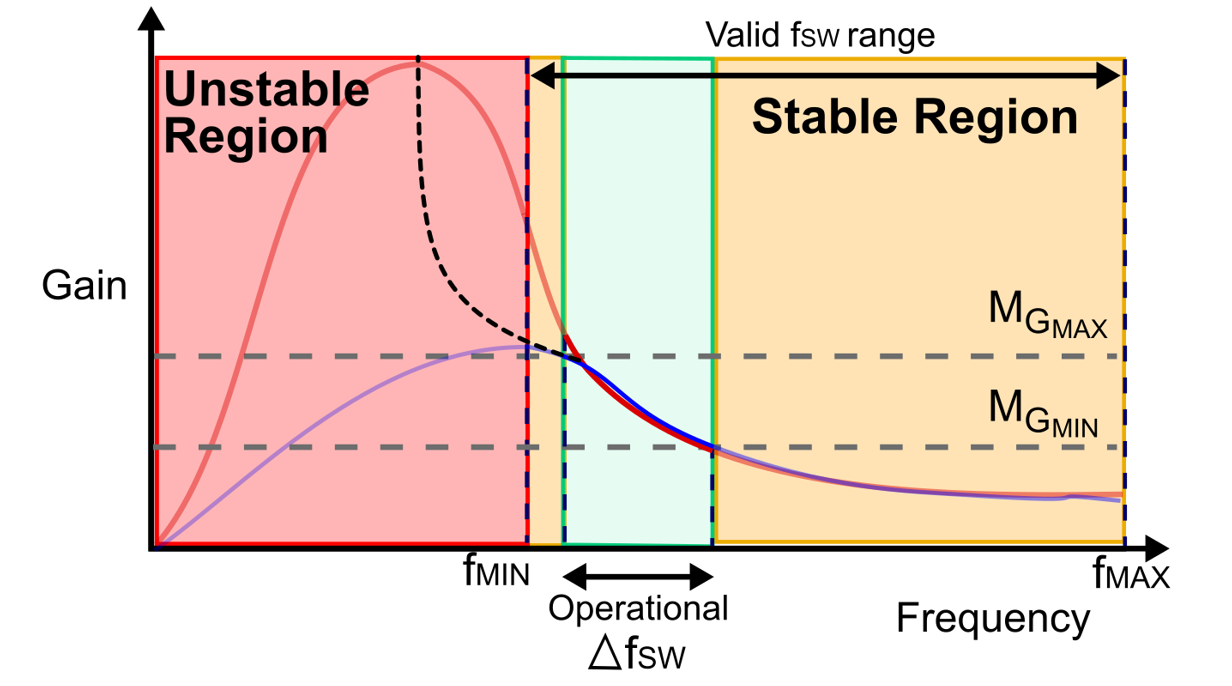## LLC 谐振腔电感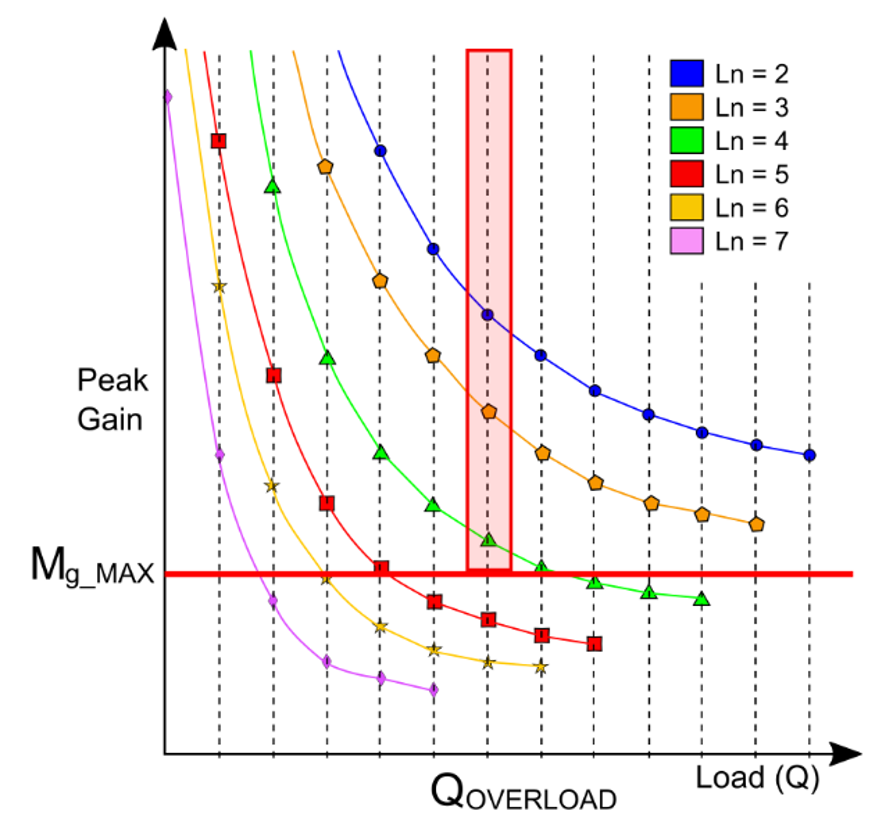## 结语

LLC 变换器的设计是一个漫长而复杂的过程，需要根据特定的应用要求考虑多种因素。由于大量的参数以及这些参数之间的关系，设计过程通常跨越多次迭代与计算，可能导致设计时间过长。而MPS 提供 LLC设计工具 以及以及可配置的LLC 控制器 MPF32010等工具，可以显著加速开发过程。